# Finding Max Element in an Array using C++

## Finding Max element in an Array using C++:

In this article, I am going to discuss the program for Finding the Max element in an Array using C++ Language with examples. Please read our previous articles, where we discussed the program for Calculating the Sum of all elements in an array using C++ Language with examples.

##### Finding Max element in an Array using C++:

Here, we will write a program for finding the maximum element in an Array using C++ Language.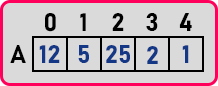Here we have an Array with some elements from which we have to find out the maximum element.

##### Procedure for Finding Max element in an Array: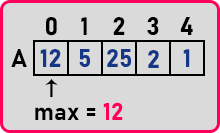Initialize the ‘max’ variable to the A element of the array. From here we will compare every element with the ‘max’ and if (A[i] > max) then we will assign max = A[i].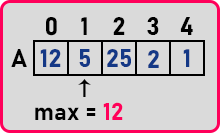Now A < max that is 5 < 12. So we will not modify max here.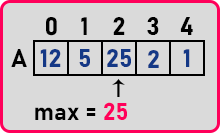Here A > max that is 25 > 12. So we will assign max to A i.e. ‘max’ = 25.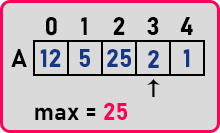Now A < max that is 2 < 25. So we will not modify max here.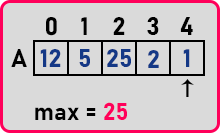Here also A < max that is 1 < 25. So we will not modify max here. After traversing the whole array, the value of ‘max’ is 25 which is the maximum element present in the array. This is a simple procedure of comparing all the elements with a single variable ‘max’. Now let us see this procedure in table form. First, we will initialize ‘max’ to A,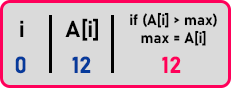Now we will compare max with A,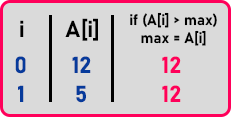‘max’ > A, so ‘max’ will remain unchanged. Then compare it with A,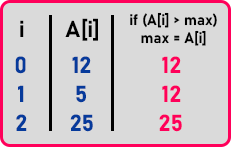A is greater than max. So we will assign max to A i.e. ‘max’ = 25. In the same manner, we will iterate through the array.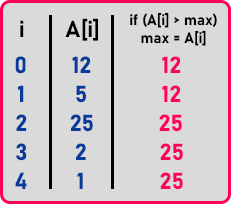We were checking that if A[i] is greater than the maximum then change Max to A[i], so we have to scan all the elements and every time check with the max. So basically, we should start from the second value onwards. So, these are the repeating steps. Let us write a program for that one.

##### Program to find maximum element in an array using C++:
```#include <iostream>
#include <conio.h>
using namespace std;
int main()
{
int n, max = 0;
cout <<"Enter size of the array: ";
cin >> n;
int A[n];
cout <<"Enter elements of the array:\n";
for (int i = 0; i < n; i++)
{
cin >> A[i];
if(i >= 1 && A[i] > max)
{
max = A[i];
}
}
cout <<"Max element : " << max << endl;
getch ();
}
```
###### Output: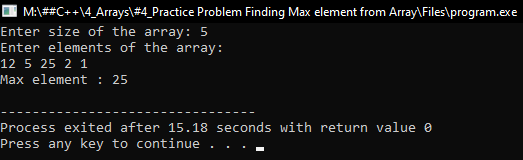### 1 thought on “Finding Max Element in an Array using C++”

1.The above code has an issue if the largest number is there at index 0 it will never be displayed.

The corrected code:-

#include
#include
using namespace std;
int main()
{
int n, max = 0;
cout <> n;
int A[n];
cout <<"Enter elements of the array:\n";
for (int i = 0; i > A[i];
if(i==0)
max = A[i];
else
{
if(i >= 1 && A[i] > max)
{
max = A[i];
}
}
}
cout <<"Max element : " << max << endl;
getch ();
}